Recent works in the field have demonstrated the superiority of the methods inspired by our brain network of neurons, and in particular the part of the brain that deals with vision and hearing, the cortex. Algorithms are now as powerful as the one found in the neocortex.

The neuron

A computer script representing a “neuron” can be shown as follow :

• inputs are the yellow boxes, and represent a signal, vision or hearing.

• coefficients are the blue boxes, modulate the signal input by a factor, and represent the dendrite

• a neuron cell computes an addition or a subtraction (inhibition). We will consider coefficient can be negative, so that this will enable to work with “addition” only.

• if the signal does not go above a threshold, the output will be transmitted. It is the A box (A for Activation).

For example, a neuron can compute an average of the input signal :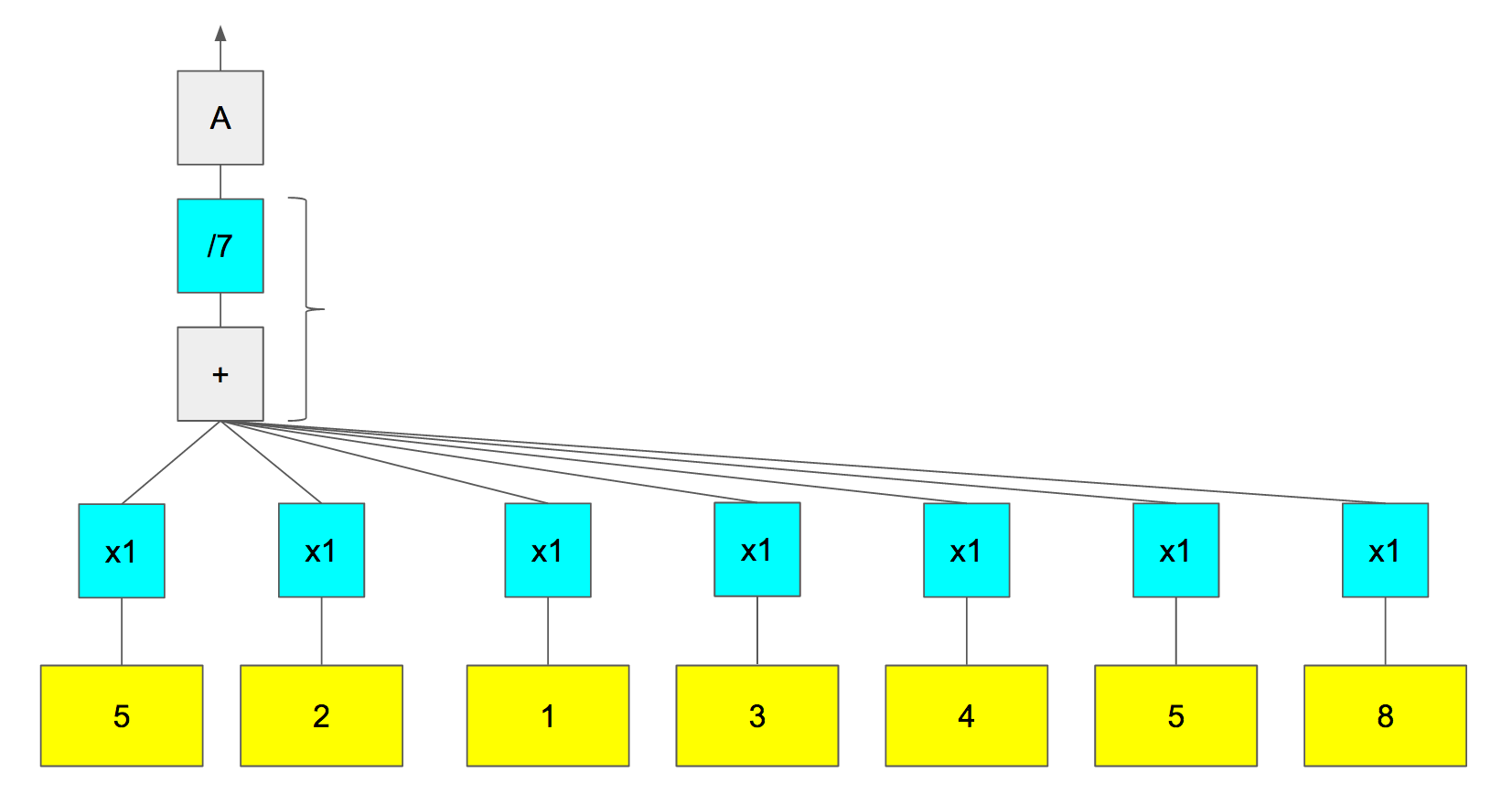The output in this case will be 4.

Computing the value of 4 by applying the operations is called propagation.

Back propagation

There is an important thing to see : if you’d like for any reason to have a “2” instead of a “4” as an output for this signal, one can follow one of these strategies :

• change the “/7” by “/14”

• change the “x1” by “/2”

• change the “x1” by “/4” for a few of them, and keep “x1” for a few other. Like the tax in a country, politics can decide to tax a bit more the rich, or a bit more the poors (because they are numerous) to get a new balance with the exact same budget.

This process of “changing the coefficients” to get the desired output value is called back-propagation.

Signal decomposition

If you remember your courses about Fourier decomposition / spectral decomposition or even more, the wavelet decomposition, you know that any signal can be decomposed as a sum of harmonics or wavelets, and the spectral amplitudes can be calculated by multiplying the inputs by the harmonics or the wavelets, which in a discrete world can be executed by multiplying the input signal by some well-chosen coefficients for a neural net :In this way, a neural network is able to perform a spectral decomposition or wavelet decomposition if we assign it the right coefficients : a neural network has the potential to capture the information from a signal.

Multi-layer, partially or full connected, and 2D

To go further in complexity, neural net’s designers usually add multiple layers of neurons :These layers are fully-connected since every neuron from previous layer is connected to every neuron of the next layer.

Some networks can be partially connected, for example applying on a kernel of size 2 :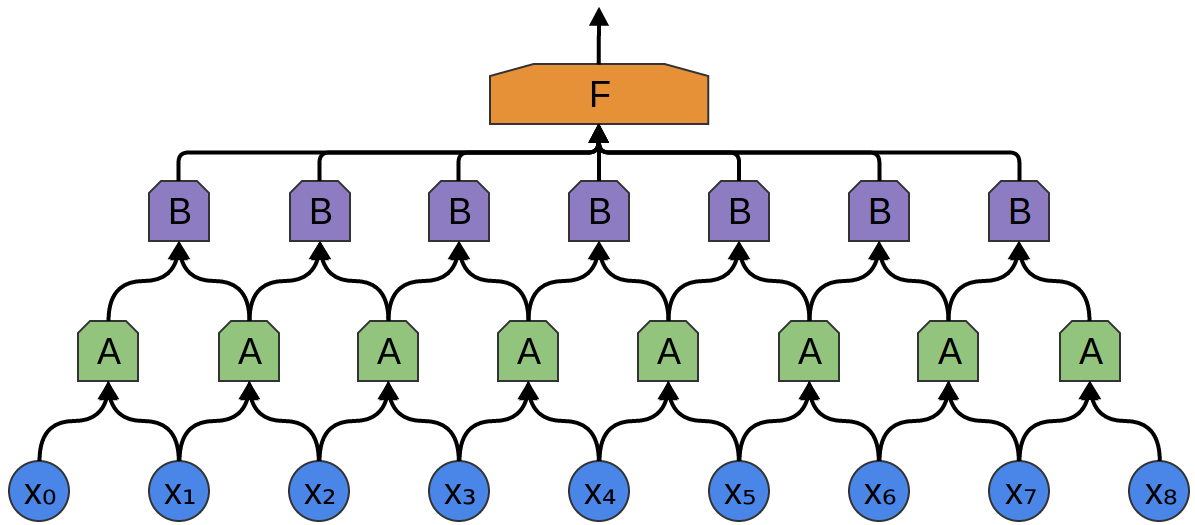http://colah.github.io/posts/2014-07-Conv-Nets-Modular/

Lastly, neural net layers can be 2D :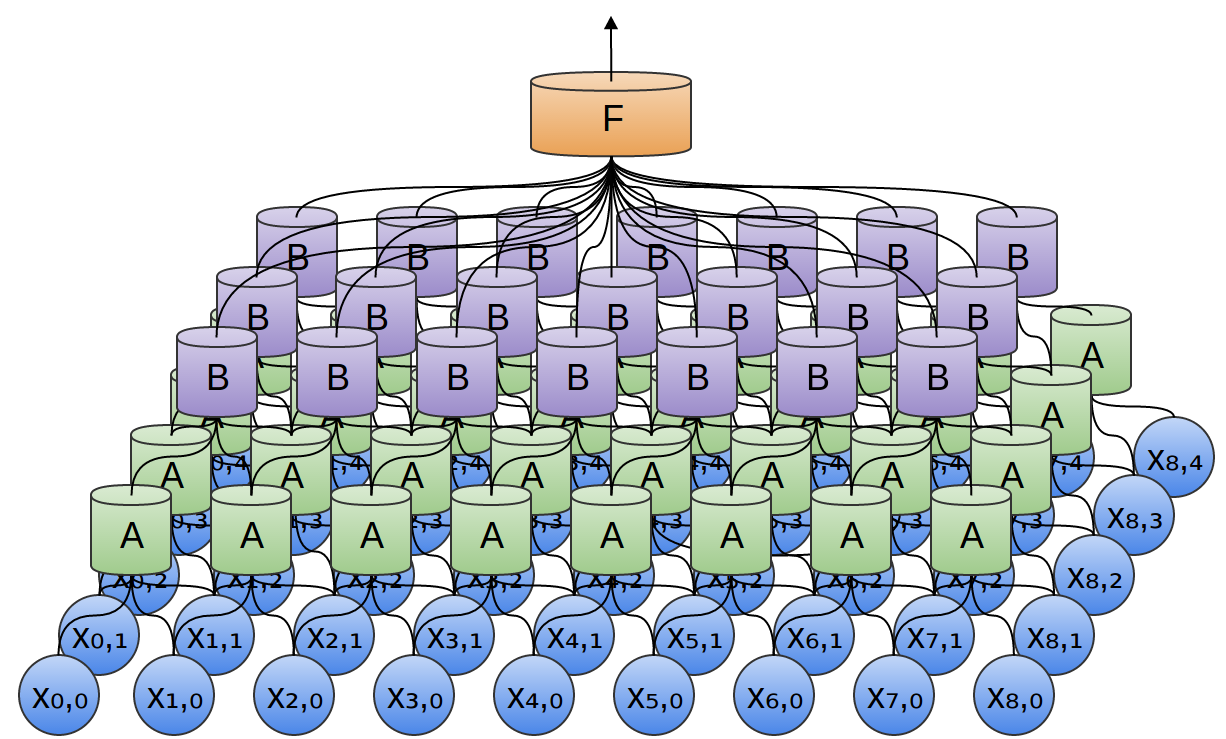http://colah.github.io/posts/2014-07-Conv-Nets-Modular/

Architecturing a neural network is quite complex and in many cases we do not know exactly why a neural network perform better than another.

Supervised learning

Learning algorithms aim at determining the right coefficients for the networks in order to be good predictors of what we want on the input signal. For example, we’d like the net to give a good prediction of an image of a “cat” to be a “cat”.

Learning is done in 2 steps :

• propagation: the training image is set as input, and the output is computed (at the beginning the network is initiated with small random values for its coefficients)

• back-propagation of the errors : if the output does not reflect the correct probability of a “cat” in the image, coefficients will be very slightly modified in a direction that will help the output to be closer to the desired probability (0 or 1).

These 2 steps are repeated many times on a big training dataset of images for which the label are known : as an example the CIFAR database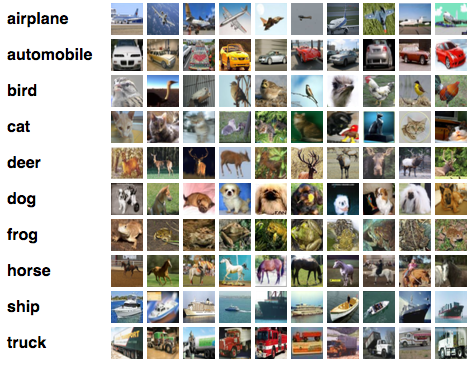That way, an “experience of the world” is transformed into “learned” coefficients in the net.

The results in classification are now so good that computers can beat non-expert humans in image classification.

When you back-propagate errors on the input image rather than on the coefficients of the net, hallucinations of objects will appear in the image, have a look :

One can consider the terrific impression of this video as a proof that neural nets are not so far from a real human brain.

Recurrent neural nets

The previous nets present a main drawback for time series and time sequences. For example, when you write a sentence, your brain remembers the subject of the sentence to conjugate the verb. It would be possible to learn all possible sentences, but the number of possible combinations is too high to be practical.

A new kind of net was introduced and gets a lot of successes, the recurrent neural nets, where the output is simply re-injected to the input of the next neural net prediction :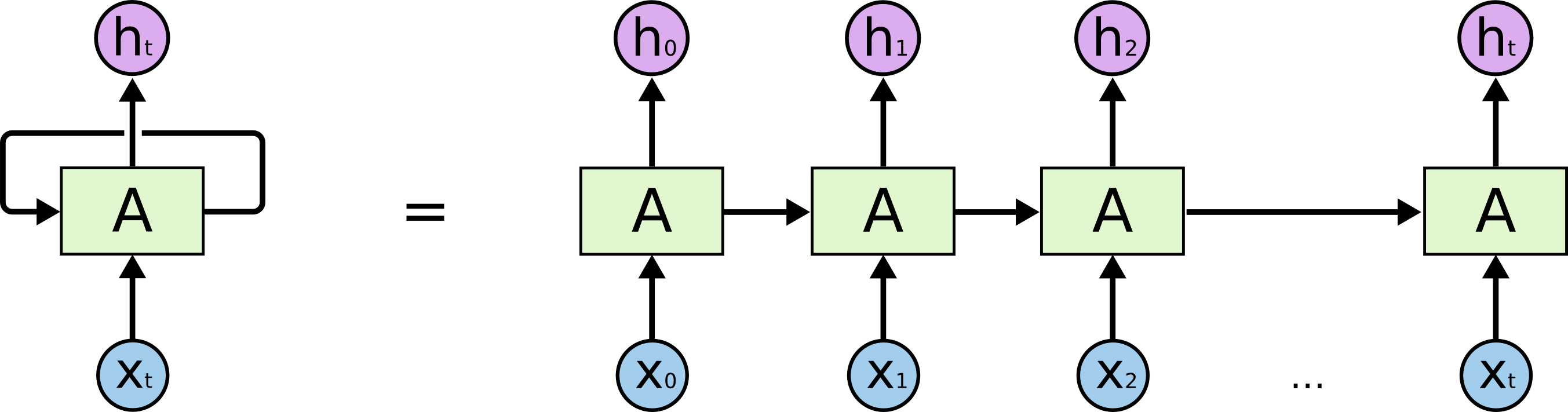http://colah.github.io/posts/2015-08-Understanding-LSTMs/

Here are very nice applications of recurrent neural nets :

• describing scenes :
• generating TED talk conferences :

We can understand from these examples that there are still missing parts, such as logic, but also emotions, attention and consciousness…

A first usage of recurrent nets for attention has been combined with spatial transformer networks :

A huge potential for the future is expected in the use of recurrent neural nets in IoT connected objects, where the past data is very important in the signal analysis to predict the correct reaction. For example, an IoT sonar device close to the bat’s echolocation system, will have to remember previously emitted sonar calls when listening to the echoes :http://deeplearning.cs.cmu.edu/notes/shaoweiwang.pdf

The new paradigm for computer programming

Computer programming consists in giving instructions to computers.

But with these new advances in artificial intelligence, some instructions can be learned by the computer itself.

So, the new programming paradigm is how to write computer programs, what should be “hard-coded” and what can be leaved to its artificial brain.

Have a look at the interview of George Hotz, who created a self-driving car with just 2000 lines of code :

Many of human brain abilities will be reproduced in artificial intelligence in the future (emotions, logic, attention, consciousness,…) and the past has demonstrated that limits about artificial intelligence possibilities have always been pushed further.